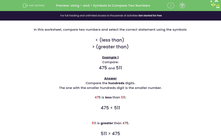# Using < and > Symbols to Compare Two Numbers

In this worksheet, students compare numbers and use the < and > symbols.Key stage:  KS 2

Curriculum topic:   Number: Number and Place Value

Curriculum subtopic:   Order/Compare Numbers to 1000

Popular topics:   Numbers worksheets

Difficulty level:#### Worksheet Overview

In this worksheet, compare two numbers and select the correct statement using the symbols:

< (less than)

> (greater than)

Example 1

Compare:

475 and 511

Compare the hundreds digits.

The one with the smaller hundreds digit is the smaller number.

475 is less than 511.

475 < 511

511 is greater than 475.

511 > 475

Example 2

Compare:

396 and 348

Compare the hundreds digits.

They are the same. So...

Compare the tens digits.

The one with the larger tens digit is the larger number.

396 is greater than 348.

396 > 348

348 is less than 396.

348 < 396

Example 3

Compare:

526 and 523

Compare the hundreds digits.

They are the same. So...

Compare the tens digits.

They are the same. So...

Compare the ones digits.

The one with the larger ones digit is the larger number.

526 is greater than 523.

526 > 523

523 is less than 526.

523 < 526

### What is EdPlace?

We're your National Curriculum aligned online education content provider helping each child succeed in English, maths and science from year 1 to GCSE. With an EdPlace account you’ll be able to track and measure progress, helping each child achieve their best. We build confidence and attainment by personalising each child’s learning at a level that suits them.

Get started